# Checkmate

#### 2023-04-26

Ever used an R function that produced a not-very-helpful error message, just to discover after minutes of debugging that you simply passed a wrong argument?

Blaming the laziness of the package author for not doing such standard checks (in a dynamically typed language such as R) is at least partially unfair, as R makes these types of checks cumbersome and annoying. Well, that’s how it was in the past.

Enter checkmate.

Virtually every standard type of user error when passing arguments into function can be caught with a simple, readable line which produces an informative error message in case. A substantial part of the package was written in C to minimize any worries about execution time overhead.

## Intro

As a motivational example, consider you have a function to calculate the faculty of a natural number and the user may choose between using either the stirling approximation or R’s factorial function (which internally uses the gamma function). Thus, you have two arguments, n and method. Argument n must obviously be a positive natural number and method must be either "stirling" or "factorial". Here is a version of all the hoops you need to jump through to ensure that these simple requirements are met:

fact <- function(n, method = "stirling") {
if (length(n) != 1)
stop("Argument 'n' must have length 1")
if (!is.numeric(n))
stop("Argument 'n' must be numeric")
if (is.na(n))
stop("Argument 'n' may not be NA")
if (is.double(n)) {
if (is.nan(n))
stop("Argument 'n' may not be NaN")
if (is.infinite(n))
stop("Argument 'n' must be finite")
if (abs(n - round(n, 0)) > sqrt(.Machine$double.eps)) stop("Argument 'n' must be an integerish value") n <- as.integer(n) } if (n < 0) stop("Argument 'n' must be >= 0") if (length(method) != 1) stop("Argument 'method' must have length 1") if (!is.character(method) || !method %in% c("stirling", "factorial")) stop("Argument 'method' must be either 'stirling' or 'factorial'") if (method == "factorial") factorial(n) else sqrt(2 * pi * n) * (n / exp(1))^n } And for comparison, here is the same function using checkmate: fact <- function(n, method = "stirling") { assertCount(n) assertChoice(method, c("stirling", "factorial")) if (method == "factorial") factorial(n) else sqrt(2 * pi * n) * (n / exp(1))^n } ## Function overview The functions can be split into four functional groups, indicated by their prefix. If prefixed with assert, an error is thrown if the corresponding check fails. Otherwise, the checked object is returned invisibly. There are many different coding styles out there in the wild, but most R programmers stick to either camelBack or underscore_case. Therefore, checkmate offers all functions in both flavors: assert_count is just an alias for assertCount but allows you to retain your favorite style. The family of functions prefixed with test always return the check result as logical value. Again, you can use test_count and testCount interchangeably. Functions starting with check return the error message as a string (or TRUE otherwise) and can be used if you need more control and, e.g., want to grep on the returned error message. expect is the last family of functions and is intended to be used with the testthat package. All performed checks are logged into the testthat reporter. Because testthat uses the underscore_case, the extension functions only come in the underscore style. All functions are categorized into objects to check on the package help page. ## In case you miss flexibility You can use assert to perform multiple checks at once and throw an assertion if all checks fail. Here is an example where we check that x is either of class foo or class bar: f <- function(x) { assert( checkClass(x, "foo"), checkClass(x, "bar") ) } Note that assert(, combine = "or") and assert(, combine = "and") allow to control the logical combination of the specified checks, and that the former is the default. ## Argument Checks for the Lazy The following functions allow a special syntax to define argument checks using a special format specification. E.g., qassert(x, "I+") asserts that x is an integer vector with at least one element and no missing values. This very simple domain specific language covers a large variety of frequent argument checks with only a few keystrokes. You choose what you like best. ## checkmate as testthat extension To extend testthat, you need to IMPORT, DEPEND or SUGGEST on the checkmate package. Here is a minimal example: # file: tests/test-all.R library(testthat) library(checkmate) # for testthat extensions test_check("mypkg") Now you are all set and can use more than 30 new expectations in your tests. test_that("checkmate is a sweet extension for testthat", { x = runif(100) expect_numeric(x, len = 100, any.missing = FALSE, lower = 0, upper = 1) # or, equivalent, using the lazy style: qexpect(x, "N100[0,1]") }) ## Speed considerations In comparison with tediously writing the checks yourself in R (c.f. factorial example at the beginning of the vignette), R is sometimes a tad faster while performing checks on scalars. This seems odd at first, because checkmate is mostly written in C and should be comparably fast. Yet many of the functions in the base package are not regular functions, but primitives. While primitives jump directly into the C code, checkmate has to use the considerably slower .Call interface. As a result, it is possible to write (very simple) checks using only the base functions which, under some circumstances, slightly outperform checkmate. However, if you go one step further and wrap the custom check into a function to convenient re-use it, the performance gain is often lost (see benchmark 1). For larger objects the tide has turned because checkmate avoids many unnecessary intermediate variables. Also note that the quick/lazy implementation in qassert/qtest/qexpect is often a tad faster because only two arguments have to be evaluated (the object and the rule) to determine the set of checks to perform. Below you find some (probably unrepresentative) benchmark. But also note that this one here has been executed from inside knitr which is often the cause for outliers in the measured execution time. Better run the benchmark yourself to get unbiased results. ### Benchmark 1: Assert that x is a flag library(checkmate) library(ggplot2) library(microbenchmark) x = TRUE r = function(x, na.ok = FALSE) { stopifnot(is.logical(x), length(x) == 1, na.ok || !is.na(x)) } cm = function(x) assertFlag(x) cmq = function(x) qassert(x, "B1") mb = microbenchmark(r(x), cm(x), cmq(x)) ## Warning in microbenchmark(r(x), cm(x), cmq(x)): less accurate nanosecond times ## to avoid potential integer overflows print(mb) ## Unit: nanoseconds ## expr min lq mean median uq max neval ## r(x) 1640 1722 11797.34 1804 1927 990314 100 ## cm(x) 1107 1189 5067.19 1230 1353 320497 100 ## cmq(x) 656 779 6072.51 779 861 467236 100 autoplot(mb) ## Coordinate system already present. Adding new coordinate system, which will ## replace the existing one.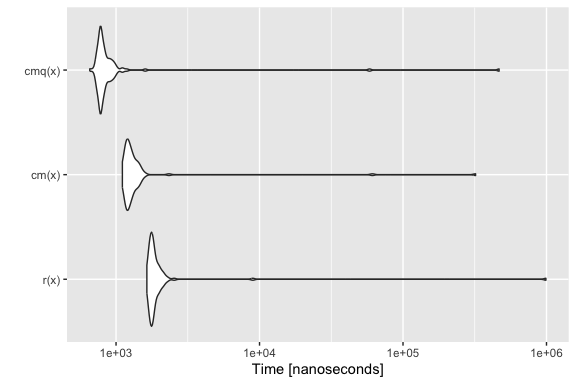### Benchmark 2: Assert that x is a numeric of length 1000 with no missing nor NaN values x = runif(1000) r = function(x) stopifnot(is.numeric(x), length(x) == 1000, all(!is.na(x) & x >= 0 & x <= 1)) cm = function(x) assertNumeric(x, len = 1000, any.missing = FALSE, lower = 0, upper = 1) cmq = function(x) qassert(x, "N1000[0,1]") mb = microbenchmark(r(x), cm(x), cmq(x)) print(mb) ## Unit: microseconds ## expr min lq mean median uq max neval ## r(x) 9.389 10.004 24.24781 10.209 10.373 1408.760 100 ## cm(x) 3.444 3.526 9.03107 3.649 3.813 475.108 100 ## cmq(x) 2.952 2.993 6.34762 3.075 3.116 324.761 100 autoplot(mb) ## Coordinate system already present. Adding new coordinate system, which will ## replace the existing one.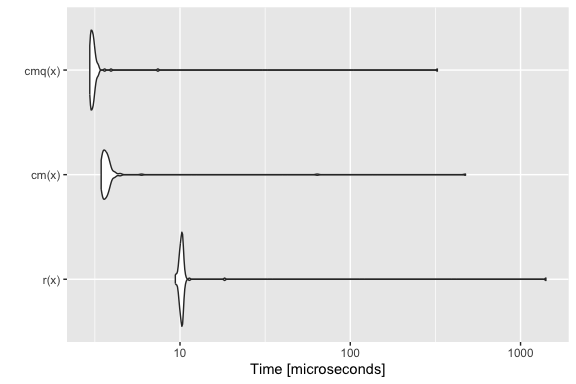### Benchmark 3: Assert that x is a character vector with no missing values nor empty strings x = sample(letters, 10000, replace = TRUE) r = function(x) stopifnot(is.character(x), !any(is.na(x)), all(nchar(x) > 0)) cm = function(x) assertCharacter(x, any.missing = FALSE, min.chars = 1) cmq = function(x) qassert(x, "S+[1,]") mb = microbenchmark(r(x), cm(x), cmq(x)) print(mb) ## Unit: microseconds ## expr min lq mean median uq max neval ## r(x) 138.457 148.1535 161.81347 150.1625 151.6795 1332.582 100 ## cm(x) 124.025 124.3120 129.90522 124.5170 124.7015 452.271 100 ## cmq(x) 58.712 58.8350 62.41635 58.9580 59.1220 380.357 100 autoplot(mb) ## Coordinate system already present. Adding new coordinate system, which will ## replace the existing one.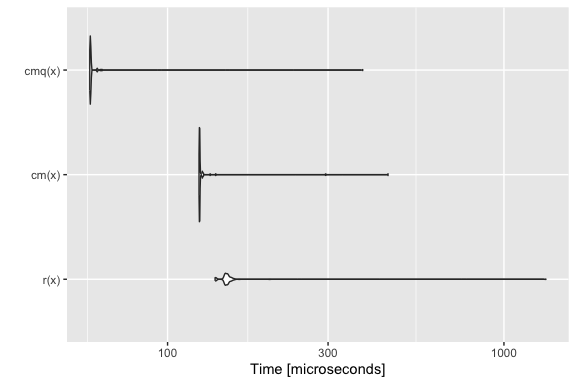### Benchmark 4: Test that x is a data frame with no missing values N = 10000 x = data.frame(a = runif(N), b = sample(letters[1:5], N, replace = TRUE), c = sample(c(FALSE, TRUE), N, replace = TRUE)) r = function(x) is.data.frame(x) && !any(sapply(x, function(x) any(is.na(x)))) cm = function(x) testDataFrame(x, any.missing = FALSE) cmq = function(x) qtest(x, "D") mb = microbenchmark(r(x), cm(x), cmq(x)) print(mb) ## Unit: microseconds ## expr min lq mean median uq max neval ## r(x) 57.277 62.771 75.28297 63.9805 65.8870 1199.496 100 ## cm(x) 22.837 23.206 28.02350 23.3290 23.7185 345.589 100 ## cmq(x) 18.819 18.983 23.58238 19.0240 19.0650 440.996 100 autoplot(mb) ## Coordinate system already present. Adding new coordinate system, which will ## replace the existing one.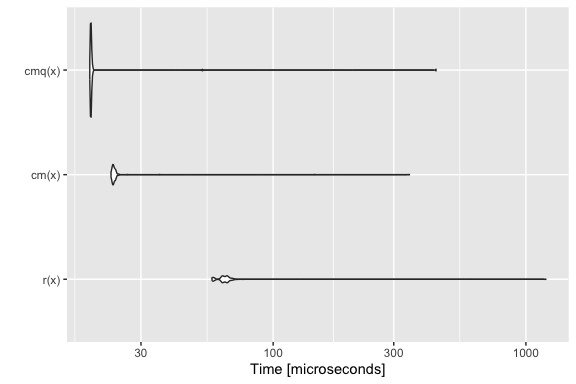# checkmate tries to stop as early as possible x$a = NA
mb = microbenchmark(r(x), cm(x), cmq(x))
print(mb)
## Unit: nanoseconds
##    expr   min      lq     mean median      uq   max neval
##    r(x) 46822 52049.5 51561.60  52316 52541.5 65477   100
##   cm(x)  2993  3198.0  3427.60   3321  3444.0 13653   100
##  cmq(x)   451   492.0   584.66    533   615.0  3690   100
autoplot(mb)
## Coordinate system already present. Adding new coordinate system, which will
## replace the existing one.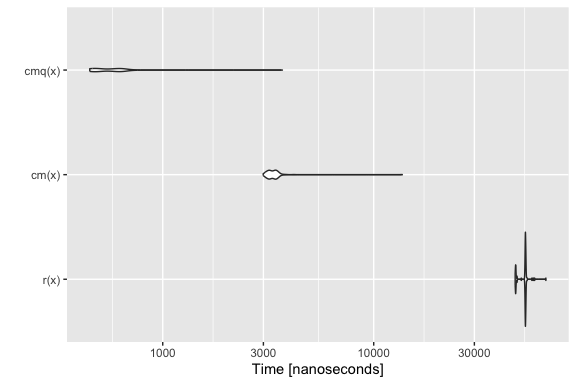### Benchmark 5: Assert that x is an increasing sequence of integers with no missing values

N = 10000
x.altrep = seq_len(N) # this is an ALTREP in R version >= 3.5.0
x.sexp = c(x.altrep)  # this is a regular SEXP OTOH
r = function(x) stopifnot(is.integer(x), !any(is.na(x)), !is.unsorted(x))
cm = function(x) assertInteger(x, any.missing = FALSE, sorted = TRUE)
mb = microbenchmark(r(x.sexp), cm(x.sexp), r(x.altrep), cm(x.altrep))
print(mb)
## Unit: microseconds
##          expr    min      lq     mean  median      uq     max neval
##     r(x.sexp) 25.092 25.2765 36.17061 26.8960 27.2445 978.096   100
##    cm(x.sexp) 10.988 11.1930 11.97323 11.3160 11.4390  74.620   100
##   r(x.altrep) 27.757 27.9825 29.24571 29.6225 30.0120  32.595   100
##  cm(x.altrep)  1.804  1.9270  6.56738  2.0500  2.2140 449.032   100
autoplot(mb)
## Coordinate system already present. Adding new coordinate system, which will
## replace the existing one.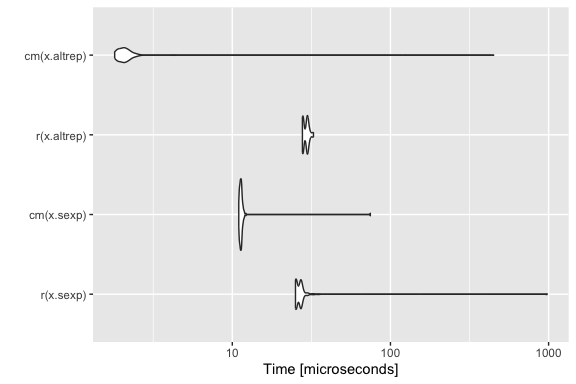## Extending checkmate

To extend checkmate a custom check* function has to be written. For example, to check for a square matrix one can re-use parts of checkmate and extend the check with additional functionality:

checkSquareMatrix = function(x, mode = NULL) {
# check functions must return TRUE on success
# and a custom error message otherwise
res = checkMatrix(x, mode = mode)
if (!isTRUE(res))
return(res)
if (nrow(x) != ncol(x))
return("Must be square")
return(TRUE)
}

# a quick test:
X = matrix(1:9, nrow = 3)
checkSquareMatrix(X)
##  TRUE
checkSquareMatrix(X, mode = "character")
##  "Must store characters"
checkSquareMatrix(X[1:2, ])
##  "Must be square"

The respective counterparts to the check-function can be created using the constructors makeAssertionFunction, makeTestFunction and makeExpectationFunction:

# For assertions:
assert_square_matrix = assertSquareMatrix = makeAssertionFunction(checkSquareMatrix)
print(assertSquareMatrix)
## function (x, mode = NULL, .var.name = checkmate::vname(x), add = NULL)
## {
##     if (missing(x))
##         stop(sprintf("argument \"%s\" is missing, with no default",
##             .var.name))
##     res = checkSquareMatrix(x, mode)
## }
# For tests:
test_square_matrix = testSquareMatrix = makeTestFunction(checkSquareMatrix)
print(testSquareMatrix)
## function (x, mode = NULL)
## {
##     isTRUE(checkSquareMatrix(x, mode))
## }
# For expectations:
expect_square_matrix = makeExpectationFunction(checkSquareMatrix)
print(expect_square_matrix)
## function (x, mode = NULL, info = NULL, label = vname(x))
## {
##     if (missing(x))
##         stop(sprintf("Argument '%s' is missing", label))
##     res = checkSquareMatrix(x, mode)
##     makeExpectation(x, res, info, label)
## }

Note that all the additional arguments .var.name, add, info and label are automatically joined with the function arguments of your custom check function. Also note that if you define these functions inside an R package, the constructors are called at build-time (thus, there is no negative impact on the runtime).

## Calling checkmate from C/C++

The package registers two functions which can be used in other packages’ C/C++ code for argument checks.

SEXP qassert(SEXP x, const char *rule, const char *name);
Rboolean qtest(SEXP x, const char *rule);

These are the counterparts to qassert and qtest. Due to their simplistic interface, they perfectly suit the requirements of most type checks in C/C++.

For detailed background information on the register mechanism, see the Exporting C Code section in Hadley’s Book “R Packages” or WRE. Here is a step-by-step guide to get you started:

1. Add checkmate to your “Imports” and “LinkingTo” sections in your DESCRIPTION file.
2. Create a stub C source file "checkmate_stub.c", see below.
3. Include the provided header file <checkmate.h> in each compilation unit where you want to use checkmate.

File contents for (2):

#include <checkmate.h>
#include <checkmate_stub.c>

## Session Info

For the sake of completeness, here the sessionInfo() for the benchmark (but remember the note before on knitr possibly biasing the results).

sessionInfo()
## R version 4.3.0 (2023-04-21)
## Platform: aarch64-apple-darwin20 (64-bit)
## Running under: macOS Ventura 13.3.1
##
## Matrix products: default
## BLAS:   /Library/Frameworks/R.framework/Versions/4.3-arm64/Resources/lib/libRblas.0.dylib
## LAPACK: /Library/Frameworks/R.framework/Versions/4.3-arm64/Resources/lib/libRlapack.dylib;  LAPACK version 3.11.0
##
## locale:
##  C/en_US.UTF-8/en_US.UTF-8/C/en_US.UTF-8/en_US.UTF-8
##
## time zone: Europe/Berlin
## tzcode source: internal
##
## attached base packages:
##  stats     graphics  grDevices utils     datasets  methods   base
##
## other attached packages:
##  microbenchmark_1.4.9 ggplot2_3.4.2        checkmate_2.2.0
##
## loaded via a namespace (and not attached):
##   vctrs_0.6.2      cli_3.6.1        knitr_1.42       rlang_1.1.0
##   xfun_0.39        highr_0.10       jsonlite_1.8.4   glue_1.6.2
##   colorspace_2.1-0 backports_1.4.1  htmltools_0.5.5  sass_0.4.5
##  fansi_1.0.4      scales_1.2.1     rmarkdown_2.21   grid_4.3.0
##  tibble_3.2.1     evaluate_0.20    munsell_0.5.0    jquerylib_0.1.4
##  fastmap_1.1.1    yaml_2.3.7       lifecycle_1.0.3  compiler_4.3.0
##  pkgconfig_2.0.3  farver_2.1.1     digest_0.6.31    R6_2.5.1
##  utf8_1.2.3       pillar_1.9.0     magrittr_2.0.3   bslib_0.4.2
##  withr_2.5.0      tools_4.3.0      gtable_0.3.3     cachem_1.0.7